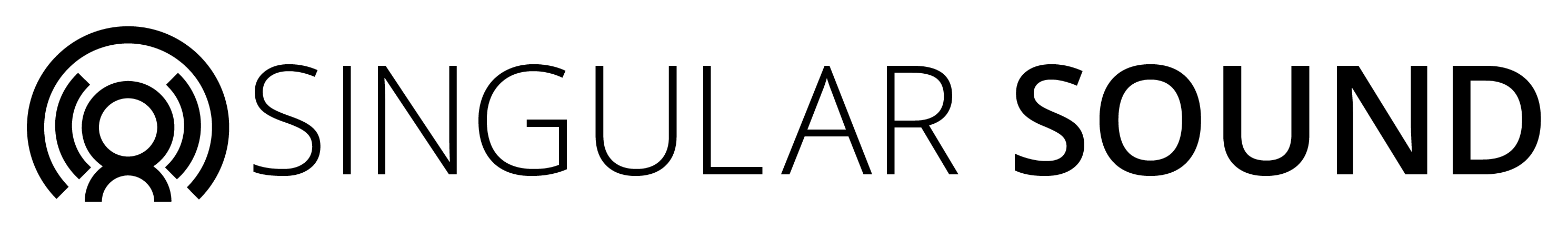# Sending Tempo change to BB?

The sending of the program change to select songs/beats in BB is fine. But can someone advise what CC (or sysex??) data is needed to send a set tempo message to the BB?

I can tell you the Hex code for 120bpm and you can adjust it for different tempos but im not sure if that is what your asking for. what device is doing the sending?

thx. I am sending MIDI data to BB from MobileSheets Pro (like onsong but for android) I want to 1. select the beat (or song if you like) and then set the tempo on a song by song basis. Might be the same beat, but with different tempos to match different songs. So if sysex is needed then please if you cna send me the string that would be appreciatedThis is the hex code I send for 120bpm
b9 6A 00
b9 6b 78
The last 2 digits on each line are the digits you can change to try out other tempos. Hope this helps

I use this in SLM to change tempo. Its enter into the raw data field

I assume you add the F0 F7 bytes as well?
so it should be F0 xx xx B9 6A 00 B9 6B 78 F7 yes?(where xx is device ID) normally the second and 3rd last bytes are the data changes IIRC??

Anyhoo, thanks it gives me a start. I might just have to load up MIDI-Ox and run some trials as well hahaha!

No not for me and my setup. I put in just what I sent to you and when that code is sent to the BB it changes the tempo to 120bpm. Did not have to add F0 or F7

oh ok, interestingTo set tempo with midi you need to send two CC messages, 106 and 107. The tempo only changes when the BB receives the 107 message.
The two messages together tell it the temp.
Think of the tempo as being made up of two numbers a+b.
If the tempo is LESS than 128 (ie up to and including 127) then ‘a’ is zero and ‘b’ is the tempo.
If tempo is 128 or more then ‘a’ is 1 and ‘b’ is the tempo-128

What it really means is “(a times 128) + b”

You send the 'a value with the 106 CC message and the ‘b’ value with the 107 CC message.

A CC message has the form (in hex):

Bn controller_number value

Where n is the channel number, eg if you want to send it on channel 1 then B code B0 (because channel numbers as coded are one less than the actual number you think of in the real world. so channel 1 becomes zero, channel 10 becomes 9 etc)

The 106 controller number becomes (in hex) 6A and the 107 CC becomes 6B.
The values are just straight conversions of the decimal values to hex (use windows calc in programmer mode to do the conversions if you cannot do them yourself). So 1 becomes 1, 127 becomes FE etc.

So for a tempo of say 130.
‘a’ becomes 1 and ‘b’ becomes 2
That is (1*128) + 2 = 130
The CC’s (assuming sending on channel 1) are then

B0 6A 01
B0 6B 02

If the tempo was say 160

‘a’ is 1 and ‘b’ is 32
That is (1*128) + 32 = 160
32 in hex is 20 so the CCs are (assuming channel 1 again)

B0 6A 01
B0 6B 20

Hope that helps